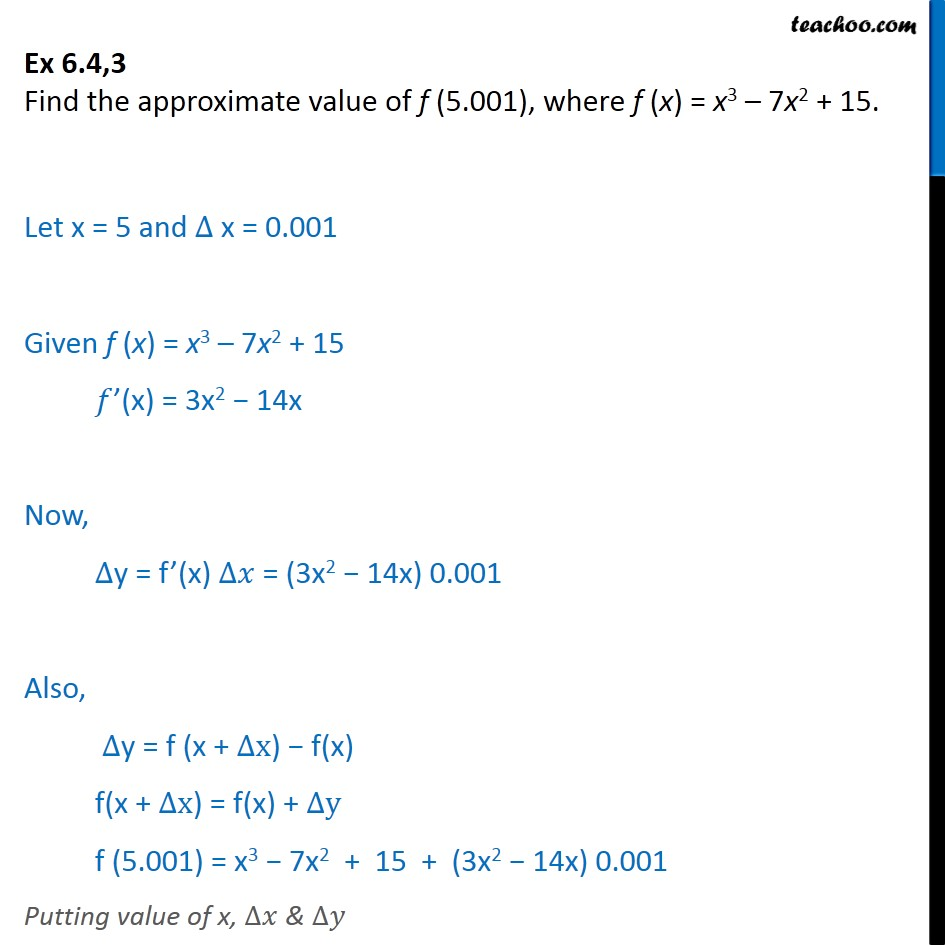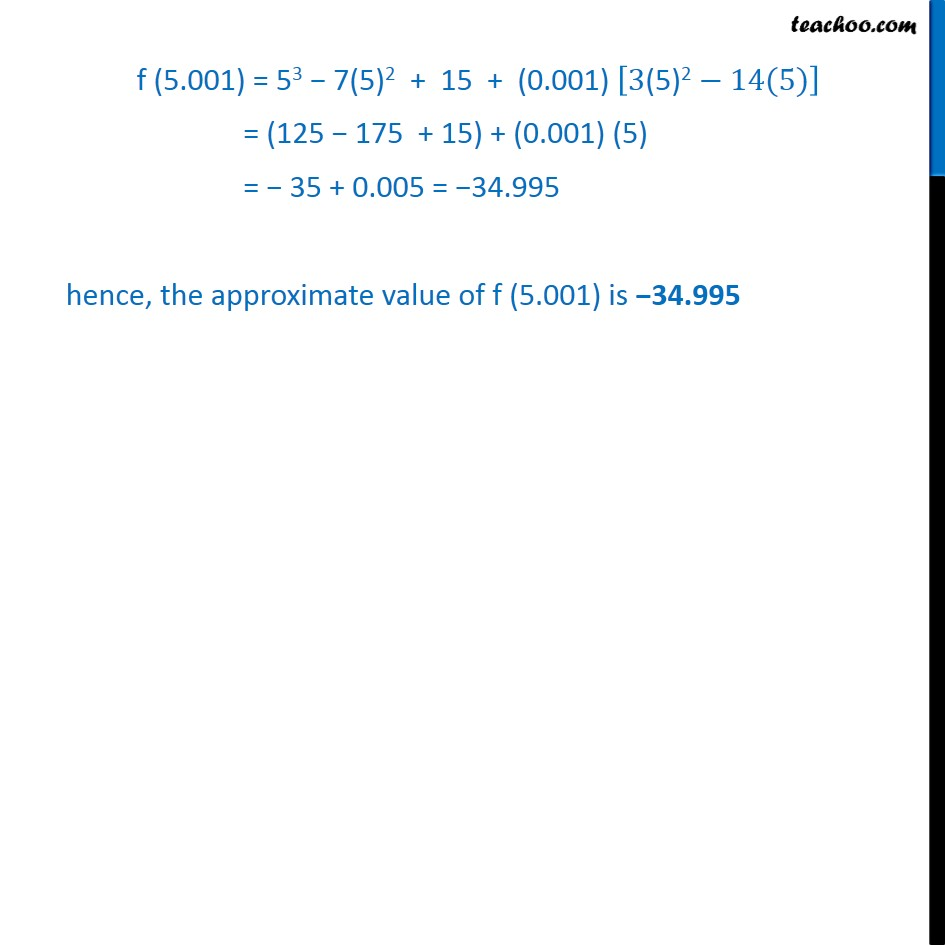1. Chapter 6 Class 12 Application of Derivatives
2. Serial order wise
3. Ex 6.4

Transcript

Ex 6.4,3 Find the approximate value of f (5.001), where f (x) = x3 7x2 + 15. Let x = 5 and x = 0.001 Given f (x) = x3 7x2 + 15 (x) = 3x2 14x Now, y = f (x) = (3x2 14x) 0.001 Also, y = f (x + x) f(x) f(x + x) = f(x) + y f (5.001) = x3 7x2 + 15 + (3x2 14x) 0.001 Putting value of x, & f (5.001) = 53 7(5)2 + 15 + (0.001) 3(5)2 14(5) = (125 175 + 15) + (0.001) (5) = 35 + 0.005 = 34.995 hence, the approximate value of f (5.001) is 34.995

Ex 6.4